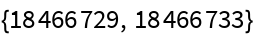#Function Repository Resource:

# CousinPrimes

Get the pairs of primes that differ by 4

Contributed by: Wolfram Staff
 ResourceFunction["CousinPrimes"][n] gives the first n pairs of primes that differ by 4.

## Examples

### Basic Examples

Here are the first 10 pairs of cousin primes:

 In:=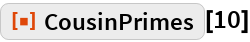Out=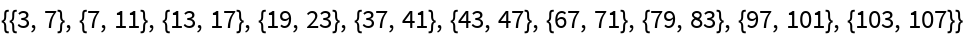Indeed, the differences are 4:

 In:=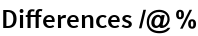Out=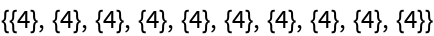### Neat Examples

The sum of the reciprocals of the primes diverges:

 In:=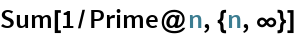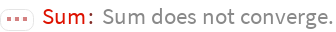Out=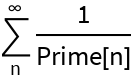The sum of the reciprocals of the cousin primes converges to a number around 1.673. Here is the sum of the reciprocals of the first 100,000 cousin primes:

 In:=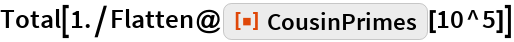Out=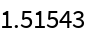This is the last pair in that sum:

 In:=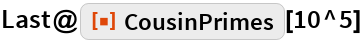Out=